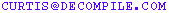# Pointer Arithmetic

###### by Curtis Krauskopf

One of the most common C++ programming problems is the way that pointers are handled in the C++ programming language.

Almost every programmer is comfortable with arrays. Code snippet 1 defines an array of 5 integers and then obtains the 4th integer in the array:

int sample;
int random = sample;

In the second line of code snippet 1, the array offset  really is the fourth integer in the array because arrays in C++ start at 0:

Array Element Array Index Value 1st  ??? 2nd  ??? 3rd  ??? 4th  ??? 5th  ???

Also, in code snippet 1, the choice of naming the variable random is not an accident or just a cute name. The value really is random because the values of the array defined in the first line of the code snippet were not defined. The result of sample in an uninitialized array is a random value -- in other words, it could be any integer value.

An array access and pointer arithmetic are the same thing. Code snippet 2 is an example of an array access. The last line of the snippet accesses the same array element using pointer arithmetic.

int sample;
int random;
random = sample;
random = *(sample + 3);

Of course, most programmers use the shorter version of those two expressions but the C++ language allows us to use either form.

Pointer arithmetic works by translating an expression:

p + n

into the following expression:

p + n * sizeof(whatever p points to)

Here's a simple example of some misleading code. The code in figure 1 is reading the values in an array of chars and then writing the value of the array elements to cout.

#include <iostream.h>

// Create a program that type casts an array of chars
// into an array of shorts and then traverse the array.
// Prevent the last 8 chars of the array from being
// dereferenced.
//
int main(int, char*)
{
const BUFFERSIZE = 20;
unsigned char *p = (unsigned char*) new char[BUFFERSIZE];
unsigned short *sptr = NULL;
int line = 0;

// Pointer arithmetic in the limit of this for() loop
// causes the wrong limit address to be calculated.
for(line = 1, sptr = (unsigned short *)p;
(sptr < (unsigned short*)p + BUFFERSIZE - 8);
sptr++, line++) {
cout << dec << "Line " << line << ": ";
cout << hex << "sptr = " << sptr << "h";
cout << ", stop at: ";
cout << ((unsigned short*)p + BUFFERSIZE - 8);
cout << "h" << dec << endl;
}

cout << "---------------------------------" << endl;

// Casting the limit part of the for() loop to an
// unsigned char* causes the program to do the right
// thing, but the displayed limit is still wrong.
for(line = 1, sptr = (unsigned short*) p;
((unsigned char*)sptr < p + BUFFERSIZE - 8);
sptr++, line++) {
cout << dec << "Line " << line << ": ";
cout << hex << "sptr = " << sptr << "h";

// --- this looks right but is wrong because
// operator<<(unsigned char*) dereferences the
// pointer passed to it and then tries to
// print what the dereferenced pointer points to:
//    cout << ", stop at: " << (p + BUFFERSIZE - 8);
//
// -- this throws a compile-time error:  size of
// type 'void' is unknown or zero
// cout << ", stop at: " << ((void*)p + BUFFERSIZE - 8);

cout << ", stop at: ";
cout << ((unsigned short*)p + BUFFERSIZE - 8);
cout << "h" << dec << endl;
}

cout << "---------------------------------" << endl;

// This is one way to do it right -- declare a variable
// to hold the for loop's limit:
unsigned char* limit = p + BUFFERSIZE - 8;
for(line = 1, sptr = (unsigned short*) p;
((unsigned char*)sptr < limit);
sptr++, line++) {
cout << dec << "Line " << line << ": ";
cout << hex << "sptr = " << sptr << "h";
// --- We can use (void*) now because we don't need to use
// any pointer arithmetic to show the right value.
cout << ", stop at: " << ((void*)limit);
cout << "h" << dec << endl;
}

return 0;
}

Popular C++ topics at The Database Managers:C++ FAQ Services | Programming | Contact Us | Recent Updates Send feedback to: